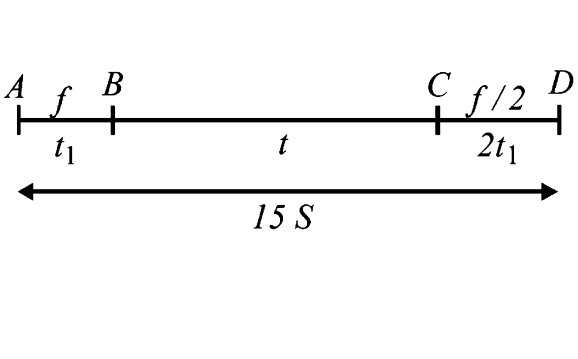# A car, starting from rest, accelerates at the rate (f) through a distance (S), then continues at constant speed for some time (t) and then decelerates

14 views
in Physics
closed
A car, starting from rest, accelerates at the rate (f) through a distance (S), then continues at constant speed for some time (t) and then decelerates at the rate  f//2 to come to rest. If the total distance is  5 S, then prove that
 S=1/2/ ft^(2).
A.  S = (1)/(6) ft^(2)
B.  S = ft
C.  s = (1)/(4) ft^(2)
D.  s = (1)/(72) ft^(2)

by (90.0k points)

Correct Answer - D
Distance from A to B = S = (1)/(2) ft_(1)^(2) rArr ft_(1)^(2) rArr ft_(1)^(2) = 2S
Distance from  B to C = (f_(1))t
Distance from C to D = (u^(2))/(2a) = ((ft_(1))^(2))/2(f//2) = ft_(1)^(2) = 2S
rArr S + f t_(1)t + 2 S = 15 S rArr f t_(1)t = 12 S
But 1/2 f t_(1)^(2) = S
On dividing the above two equations , we get t_(1) = (t)/(6)
rArr S = 1/2 f (t/6)^(2) = (f t^(2))/(72)